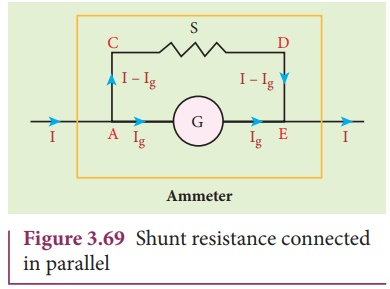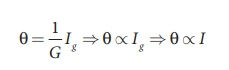Home | | Physics 12th Std | Conversion of galvanometer into ammeter and voltmeter

# Conversion of galvanometer into ammeter and voltmeter

A galvanometer is very sensitive instrument to detect the current. It can be easily converted into ammeter and voltmeter.

Conversion of galvanometer into ammeter and voltmeter

A galvanometer is very sensitive instrument to detect the current. It can be easily converted into ammeter and voltmeter.

## Galvanometer to an Ammeter

Ammeter is an instrument used to measure current flowing in the electrical circuit. The ammeter must offer low resistance such that it will not change the current passing through it. So ammeter is connected in series to measure the circuit current.

A galvanometer is converted into an ammeter by connecting a low resistance in parallel with the galvanometer. This low resistance is called shunt resistance S. The scale is now calibrated in ampere and the range of ammeter depends on the values of the shunt resistance.Let I be the current passing through the circuit as shown in Figure 3.69. When current I reaches the junction A, it divides into two components. Let Ig be the current passing through the galvanometer of resistance Rg through a path AGE and the remaining current (I – Ig) passes along the path ACDE through shunt resistance S. The value of shunt resistance is so adjusted that current Ig produces full scale deflection in the galvanometer. The potential difference across galvanometer is same as the potential difference across shunt resistance.Since, the deflection in the galvanometer is proportional to the current passing through it.So, the deflection in the galvanometer measures the current I passing through the circuit (ammeter).

Shunt resistance is connected in parallel to galvanometer. Therefore, resistance of ammeter can be determined by computing the effective resistance, which isSince, the shunt resistance is a very low resistance and the ratio S/ Rg is also small. This means, Rg is also small, i.e., the resistance offered by the ammeter is small. So, when we connect ammeter in series, the ammeter will not change the resistance appreciably and also the current in the circuit. For an ideal ammeter, the resistance must be equal to zero. Hence, the reading in ammeter is always lesser than the actual current in the circuit. Let Iideal be current measured from ideal ammeter and Iactual be the actual current measured in the circuit by the ammeter.Then, the percentage error in measuring a current through an ammeter is### Key points

1. An ammeter is a low resistance instrument and it is always connected in series to the circuit

2. An ideal ammeter has zero resistance

3. In order to increase the range of an ammeter n times, the value of shunt resistance to be connected in parallel is S =G/n-1## Galvanometer to a voltmeter

A voltmeter is an instrument used to measure potential difference across any two points in the electrical circuits. It should not draw any current from the circuit otherwise the value of potential difference to be measured will change.

Voltmeter must have high resistance and when it is connected in parallel, it will not draw appreciable current so that it will indicate the true potential difference.A galvanometer is converted into a voltmeter by connecting high resistance Rh in series with galvanometer as shown in Figure 3.74. The scale is now calibrated in volt and the range of voltmeter depends on the values of the resistance connected in series i.e. the value of resistance is so adjusted that only current Ig produces full scale deflection in the galvanometer.

Let Rg be the resistance of galvanometer and Ig be the current with which the galvanometer produces full scale deflection. Since the galvanometer is connected in series with high resistance, the current in the electrical circuit is same as the current passing through the galvanometer.Since the galvanometer and high resistance are connected in series, the total resistance or effective resistance gives the resistance of voltmeter. The voltmeter resistance is

Rv = Rg +Rh

Therefore,Note that    I g V

The deflection in the galvanometer is proportional to current Ig. But current Ig is proportional to the potential difference. Hence the deflection in the galvanometer is proportional to potential difference. Since the resistance of voltmeter is very large, a voltmeter connected in an electrical circuit will draw least current in the circuit. An ideal voltmeter is one which has infinite resistance.

### Key points

1.     Voltmeter is a high resistance instrument and it is always connected in parallel with the circuit element across which the potential difference is to be calculated

2.     An ideal voltmeter has infinite resistance

3. In order to increase the range of voltmeter n times the value of resistance to be connected in series with galvanometer is R = (n-1) G

Study Material, Lecturing Notes, Assignment, Reference, Wiki description explanation, brief detail
12th Physics : Magnetism and Magnetic Effects of Electric Current : Conversion of galvanometer into ammeter and voltmeter |